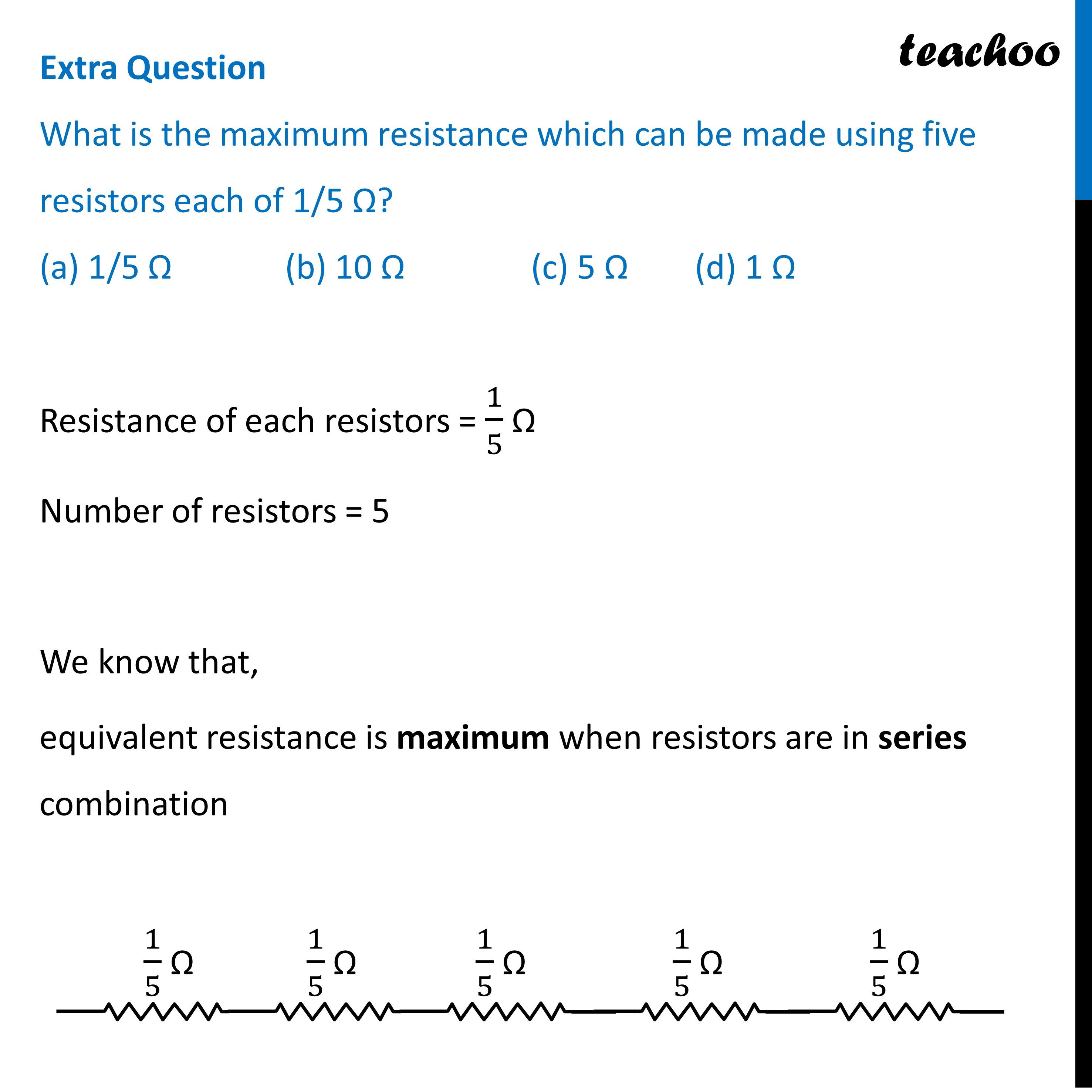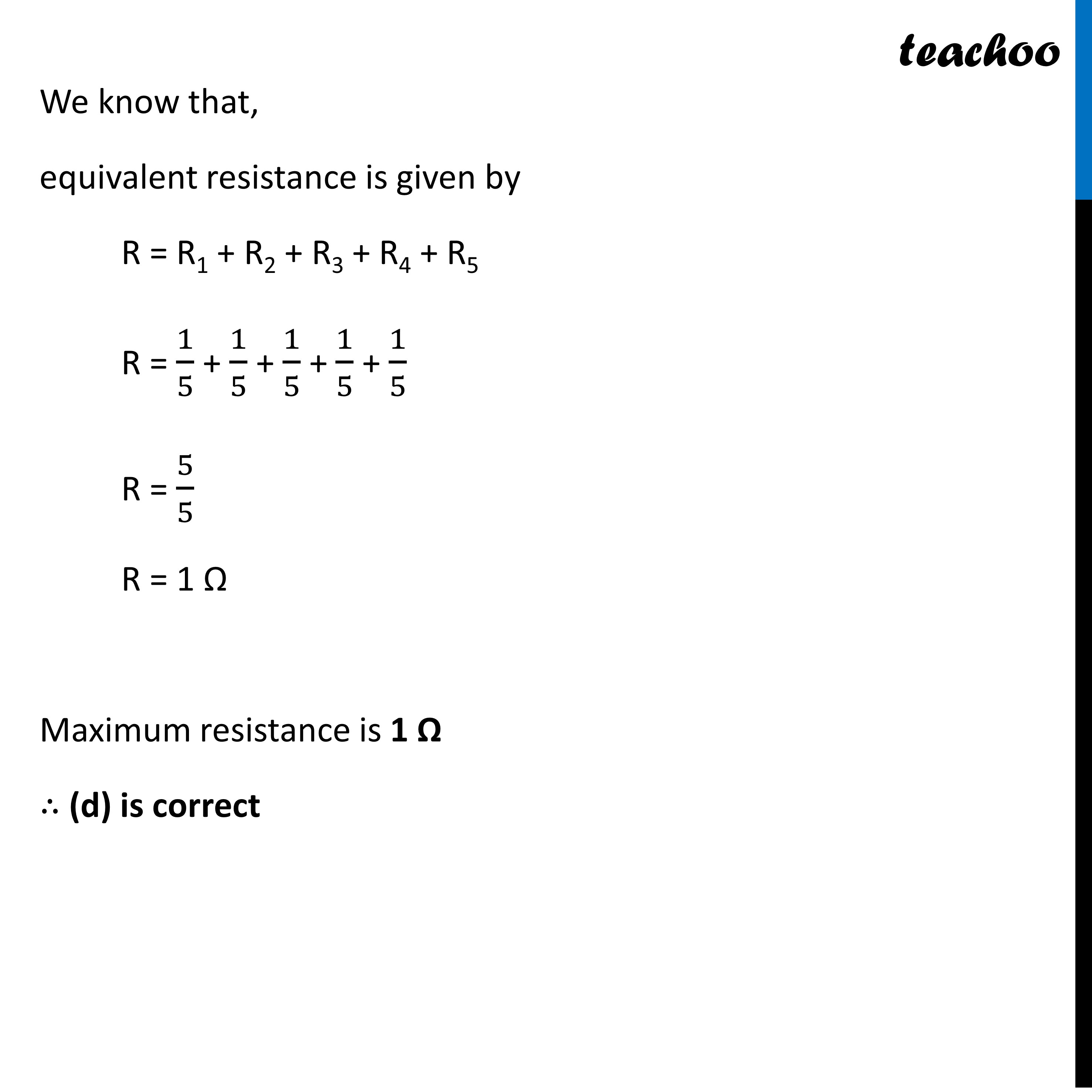MCQs from (Past Year Papers & NCERT Exemplar)

Class 10
Chapter 12 Class 10 - Electricity

## (d) 1 ΩLearn in your speed, with individual attention - Teachoo Maths 1-on-1 Class

### Transcript

Extra Question What is the maximum resistance which can be made using five resistors each of 1/5 Ω? (a) 1/5 Ω (b) 10 Ω (c) 5 Ω (d) 1 ΩResistance of each resistors = 1/5 Ω Number of resistors = 5 We know that, equivalent resistance is maximum when resistors are in series combination We know that, equivalent resistance is given by R = R1 + R2 + R3 + R4 + R5 R = 1/5 + 1/5 + 1/5 + 1/5 + 1/5 R = 5/5 R = 1 Ω Maximum resistance is 1 Ω ∴ (d) is correct